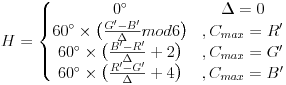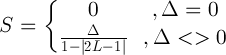# RGB and HSL

#### Wed Nov 9, 2016

##### 1157 Words

Recently my teacher Almir Olivette Artero, gave us a work to convert RGB to HSL or vice-versa to start to learn a little about CG.

Then, I decided to share here how I did the calculation and implementation.

Notice that it is possible to visualize with the result the preview of the color.

Lastly, I will post in another post, how to manipulate images showing all code used.

## HSL to RGB

Second Wikipedia, HSL are the two most common cylindrical-coordinate representations of points in an RGB color model. The representation rearrange the geometry of RGB in an attempt to be more intuitive and perceptually relevant than the cartesian (cube) representation. Developed in the 1970s for computer graphics applications, HSL are used today in color pickers, in image editing software, and less commonly in image analysis and computer vision.

HSL stands hue, saturation and lightness, where hue is defined by angular value between 0° and 360°. Starting by red at 0°, passing through the green primary at 120° and the blue primary at 240°, and then going back to red at 360°. Another two properties saturation and lightness is defined between 0% and 100%, where lightness from black to white and saturation is about gray colors scales to the definitive color.

To calculate, we used the formula where hue is defined 0 ≤ H < 360, saturation 0 ≤ S ≤ 1 and lightness 0 ≤ L ≤ 1 values.

Then we defined the following variables:

C = (1 - |2L - 1|) × S

X = C × (1 - |(H / 60°) mod 2 - 1|)

m = L - C/2Finally, with this variables, we can do the following calculation to get RGB through R’G’B’ values:

(R,G,B) = ((R’+m)×255, (G’+m)×255,(B’+m)×255)

Using this formula, we can test with the form below implemented with Javascript:

Result HSL to RGB

# Algorithm Implemented in Javascript

Obs: the code already verify input values :)

 `````` 1 2 3 4 5 6 7 8 9 10 11 12 13 14 15 16 17 18 19 20 21 22 23 24 25 26 27 28 29 30 31 32 33 34 35 36 37 38 39 40 41 42 43 44 45 46 47 48 49 50 51 52 53 54 55 56 57 58 59 60 61 62 63 64 65 66 67 68 `````` `````` function hslToRGB() { h = document.getElementById('h').value; s = document.getElementById('s').value; l = document.getElementById('l').value; if( h=="" ) h=0; if( s=="" ) s=0; if( l=="" ) l=0; h = parseFloat(h); s = parseFloat(s); l = parseFloat(l); if( h<0 ) h=0; if( s<0 ) s=0; if( l<0 ) l=0; if( h>=360 ) h=359; if( s>100 ) s=100; if( l>100 ) l=100; s/=100; l/=100; C = (1-Math.abs(2*l-1))*s; hh = h/60; X = C*(1-Math.abs(hh%2-1)); r = g = b = 0; if( hh>=0 && hh<1 ) { r = C; g = X; } else if( hh>=1 && hh<2 ) { r = X; g = C; } else if( hh>=2 && hh<3 ) { g = C; b = X; } else if( hh>=3 && hh<4 ) { g = X; b = C; } else if( hh>=4 && hh<5 ) { r = X; b = C; } else { r = C; b = X; } m = l-C/2; r += m; g += m; b += m; r *= 255.0; g *= 255.0; b *= 255.0; r = Math.round(r); g = Math.round(g); b = Math.round(b); console.log(r); console.log(g); console.log(b); } ``````

## RGB to HSL

Continuing with RGB to HSL, second Wikipédia, The RGB color model is an additive color model in which red, green and blue light are added together in various ways to reproduce a broad array of colors. The name of the model comes from the initials of the three additive primary colors, red, green and blue. The main purpose of the RGB color model is for the sensing, representation and display of images in electronic systems, such as televisions and computers, though it has also been used in conventional photography. Before the electronic age, the RGB color model already had a solid theory behind it, based in human perception of colors.

The intensity each color is defined with values between 0 and 255. For quick example, if we want the pure red, we can define in RGB: (255, 0, 0).

So, let’s start with the math formula implemented in JavaScript:

Variables:

R’ = R/255

G’ = G/255

B’ = B/255

Cmax = max(R’, G’, B’)

Cmin = min(R’, G’, B’)

Δ = Cmax - Cmin

With these variables, we used the following formula to get Hue, Saturation and Lightness respectly:

# Hue# Saturation# Lightness

L = (Cmax + Cmin) / 2

And now, we can test this formula:

Result RGB to HSL

# Algorithm Implemented in Javascript

Obs: the code already verify input values :)

 `````` 1 2 3 4 5 6 7 8 9 10 11 12 13 14 15 16 17 18 19 20 21 22 23 24 25 26 27 28 29 30 31 32 33 34 35 36 37 38 39 40 41 42 `````` `````` function rgbToHSL() { r = document.getElementById('r').value; g = document.getElementById('g').value; b = document.getElementById('b').value; if( r=="" ) r=0; if( g=="" ) g=0; if( b=="" ) b=0; r = parseFloat(r); g = parseFloat(g); b = parseFloat(b); if( r<0 ) r=0; if( g<0 ) g=0; if( b<0 ) b=0; if( r>255 ) r=255; if( g>255 ) g=255; if( b>255 ) b=255; r/=255; g/=255; b/=255; M = Math.max(r,g,b); m = Math.min(r,g,b); d = M-m; if( d==0 ) h=0; else if( M==r ) h=((g-b)/d)%6; else if( M==g ) h=(b-r)/d+2; else h=(r-g)/d+4; h*=60; if( h<0 ) h+=360; l = (M+m)/2; if( d==0 ) s = 0; else s = d/(1-Math.abs(2*l-1)); s*=100; l*=100; console.log(h.toFixed(0)); console.log(s.toFixed(1)); console.log(l.toFixed(1)); } ``````

## Conclusion

The two color systems have come to revolutionize color representation in the electronic age. It’s pretty excity to learn about how the colors in the human eye works and how we can represent images in pixels, by mixing colors represented by bits. I really hope to post more things about CG in this blog. If you liked this post, found some error or something I can do better, please comment ! :)